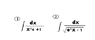# An integral problem

• transgalactic
In summary, the first integral can be solved by using partial fractions, while the second integral can be solved by making a substitution and using the arctan function.

#### transgalactic

i tried to solve these integral by substitution
in the first integral i substituted x^2 for t

in the second integral i substituted e^x

it didnt work

how to solve these integrals?

here is the file

here is the file

#### Attachments

•ggg2.GIF
1,016 bytes · Views: 502
mm i think you must use fraction partial ...and in denominator adding +1 and - 1 . to use fraction partial .

1/(x^4 +1 +1 -1 ) = 1/(x^4 - 1 )+2

1/[(x^2-1)(x^2+1)+2]

1/[(x-1)(x+1)((x^2 -1)+2) +2]

1/[(x-1)(x+1)((x-1)(x+1)+2)+2]

and you can use this web http://integrals.wolfram.com/index.jsp

x.users said:
mm i think you must use fraction partial ...and in denominator adding +1 and - 1 . to use fraction partial .

1/(x^4 +1 +1 -1 ) = 1/(x^4 - 1 )+2

1/[(x^2-1)(x^2+1)+2]

1/[(x-1)(x+1)((x^2 -1)+2) +2]

1/[(x-1)(x+1)((x-1)(x+1)+2)+2]

and you can use this web http://integrals.wolfram.com/index.jsp

and

and respect to second probelm

int[1/(e^x - 1)]

put 1 = e^x/e^x

its become int[ e^x/(e^2x - e^x)]

put u = e^x subtitution

du = e^x dx

du = u dx

so dx = du/u

int [u du/(u^2 - u ) u]

and u =! 0 because u = e^x and exponantioal never will be zero

int[du/u(u-1)] its fraction partial

First one is not fun at all, try x.users way.

For your second one, its a simple substitution.

$$u=\sqrt{e^x-1}$$

$$dx=\frac{2\sqrt{e^x-1}}{e^x} du = \frac{2u}{u^2+1} du$$

So $$\int \frac{1}{\sqrt{e^x-1}} dx$$ becomes

$$\int \frac{1}{u} \frac{2u}{u^2+1} du$$.

u's cancel out, take out the factor of 2.

$$2\int \frac{1}{1+u^2} du$$.

That integral is easy, arctan.

$$\int \frac{1}{\sqrt{e^x-1}} dx = 2\arctan (\sqrt{e^x-1})$$

x.users said:
1/[(x-1)(x+1)((x-1)(x+1)+2)+2]

and you can use this web http://integrals.wolfram.com/index.jsp
How can you Partial Fraction this?
$$\frac{1}{(x - 1) (x + 1) ((x - 1)(x + 1) + 2) + 2}$$?For the first one, you can try the following:

$$\int \frac{dx}{x ^ 4 + 1} = \frac{1}{2} \int \frac{(x ^ 2 + 1) - (x ^ 2 - 1)}{x ^ 4 + 1} dx = \frac{1}{2} \left( \int \frac{x ^ 2 + 1}{x ^ 4 + 1} dx + \int \frac{x ^ 2 - 1}{x ^ 4 + 1} dx \right) = \frac{1}{2} (I_1 + I_2)$$

$$I_1 = \int \frac{x ^ 2 + 1}{x ^ 4 + 1} dx = \int \frac{1 + \frac{1}{x ^ 2}}{x ^ 2 + \frac{1}{x ^ 2}} dx = \int \frac{1 + \frac{1}{x ^ 2}}{\left( x - \frac{1}{x} \right) ^ 2 + 2} dx$$

Now, make the u-substitution: $$u = x - \frac{1}{x} \Rightarrow du = \left(1 + \frac{1}{x ^ 2} \right) dx$$, your integral will become:

$$I_1 = \int \frac{du}{u ^ 2 + (\sqrt{2}) ^ 2} = \frac{1}{\sqrt{2}} \arctan \left( \frac{u}{\sqrt{2}} \right) + C_1 = \frac{1}{\sqrt{2}} \arctan \left( \frac{x + \frac{1}{x}}{\sqrt{2}} \right) + C_1$$

The second integral can be done by the u-substitution: $$u = x + \frac{1}{x}$$

$$I_2 = \int \frac{x ^ 2 - 1}{x ^ 4 + 1} dx = \int \frac{1 - \frac{1}{x ^ 2}}{x ^ 2 + \frac{1}{x ^ 2}} dx = \int \frac{1 - \frac{1}{x ^ 2}}{\left( x + \frac{1}{x} \right) ^ 2 - 2} dx = ...$$
Can you go from here? :)

Last edited:
thank alot!